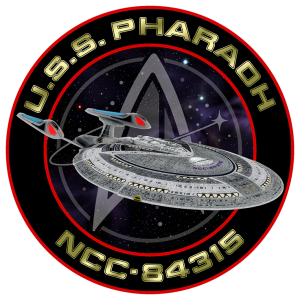######## DISTANCE:

1 Light year = 189 090 000 000 KM
1 Parsec = 3.26 Light years

##### DISTANCE BETWEEN:

Earth and Moon = 400000 KM
Earth’s sun and Pluto = 6 Billion KM
Sector 001 and centre of galaxy = 3100 LY
Standard planetary orbit = 35000 KM
Diameter of Milky Way Galaxy = 100000 LY / 30675 Parsecs

##### STARFLEET TIME STANDARDS:

1 Starfleet duty shift = 8 h = 3.3 Stardate units
10 Stardate units = 24 h = 1 Starfleet day

##### SPEED:

Standard orbit = 9600 KM per hour
Standard impulse = 0.25 x Speed of light
Maximum impulse = 0.92 x Speed of light
Speed of light = 299793 KM per second = 186000 Miles per second
Subspace radio = Warp factor 9.9999

[Revised 2.2389]